### TIME SERIES WITH VTI:

The Vanguard Total Stock Market ETF (NYSEARCA: VTI) tracks the performance of the CRSP U.S. Total Market Index. The fund has returned 6.23% since its inception in 2001. The fund is a market capitalization-weighted index that measures the entire investable U.S. equities market. It includes small-, mid- and large-cap companies. The fund is managed in a passive manner and uses an index-sampling strategy.

library(quantmod)
require(RCurl)
require(foreign)
x <- getURL("https://raw.githubusercontent.com/RInterested/datasets/gh-pages/VTI%20historical%20data.csv")
VTI <- read.csv(text = x, sep =",")
VTI$Date <- as.Date(VTI$Date, format = "%Y-%m-%d")
rownames(VTI) <- VTI$Date VTI$Date <- NULL                   # Removing the Date column
VTI <- as.xts(VTI)
chartSeries(VTI, type="auto", theme=chartTheme('white'), yrange = c(0,180))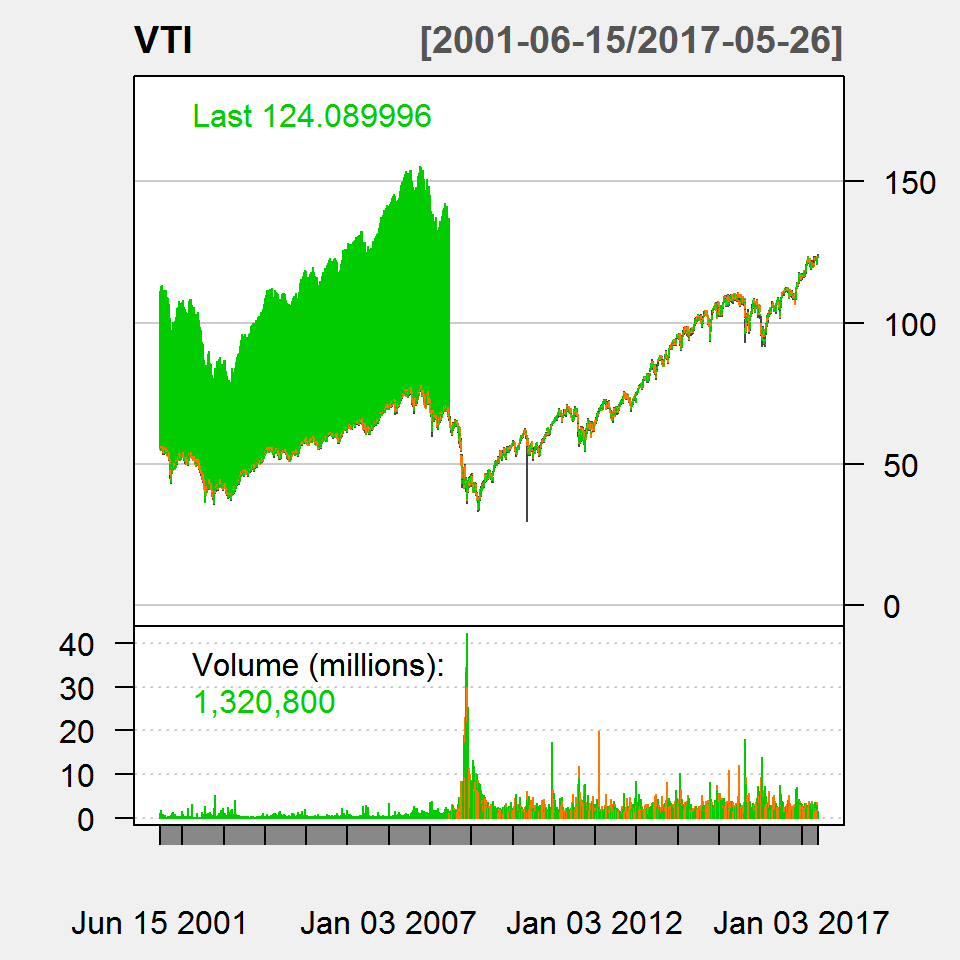Notice the split of the shares reflected as the broad band on the left of the plot:

Vanguard Total Stock Market ETF (VTI) has $$1$$ split in our VTI split history database. The split for VTI took place on June $$18$$, 2008. This was a $$2$$ for $$1$$ split, meaning for each share of VTI owned pre-split, the shareholder now owned $$2$$ shares.

##### How much can the VTI shares oscillate from day to day?
z = as.data.frame(VTI$Adj.Close) # Subsetting closing price # Function to calculate % change in closing price between days: D2D = function (x) { days = nrow(x) delta = numeric(days) for(i in 2:days){ delta[i] <- (100*((x[i,1] - x[i - 1,1])/(x[i - 1,1]))) } delta } VTI$InterDay = D2D(z)               # Included as add'l column to VTI.

head(VTI$InterDay) # A zero in entry 1 spells trouble... ## InterDay ## 2001-06-15 0.0000000 ## 2001-06-18 -0.6377436 ## 2001-06-19 0.2802368 ## 2001-06-20 1.0547174 ## 2001-06-21 0.8028564 ## 2001-06-22 -0.6371699 #... we need something to fill in the 0 in row 1. Why not the 2nd value? VTI$InterDay<-VTI$InterDay summary(VTI$InterDay)
##      Index                        InterDay
##  Min.   :2001-06-15 00:00:00   Min.   :-9.35295
##  1st Qu.:2005-06-14 18:00:00   1st Qu.:-0.47824
##  Median :2009-06-09 12:00:00   Median : 0.07394
##  Mean   :2009-06-08 08:19:59   Mean   : 0.02713
##  3rd Qu.:2013-06-04 06:00:00   3rd Qu.: 0.56837
##  Max.   :2017-05-26 00:00:00   Max.   :12.82977

So the maximum loss between days has been $$-9.4\%$$, and the maximum gain $$12.8\%$$. When did these events occur?

time(VTI)[VTI$InterDay == min(VTI$InterDay)]
##  "2008-10-15 EDT"
time(VTI)[which.max(VTI$InterDay)] ##  "2008-10-13 EDT" … both during the financial crisis of 2008. How often does a drop of say, $$-4\%$$ occurs? sum(VTI$InterDay < -4)
##  28
mean(VTI$InterDay < -4) * 100 # or 0.7% of the days ##  0.6979063 sum(VTI$InterDay < -4) / 16   # or between 1 and 2 days every year.
##  1.75

… and what about gains of $$4\%$$?

sum(VTI$InterDay > 4) ##  23 mean(VTI$InterDay > 4) * 100 # or 0.6% of the days
##  0.5732802
sum(VTI$InterDay > 4) / 16 # or between 1 and 2 days every year? ##  1.4375 But they can’t be distributed uniformly… Let’s first look at the losing days (to the left), and compare to winning days: par(mfrow=c(1,2)) plot(time(VTI), VTI$InterDay < -4, col=2, type='h', xaxt="n",
xlab="Year", ylab="Days with > - 4% change",
cex.axis=.5, cex.main=.8, cex.lab =.5,las=2,
main  = "Clustering of big drop days")

# Selecting the time information in the names of the rows of VTI:
tt = time(VTI)
# We select points spaced by approximately 1 business year:
# 365 days - 2 days off each weekend - 9 Holidays in the USA
ix = seq(1, nrow(VTI), by = 365 - 2*4*12 - 9)
# Formatting the labels as just simply the year with 4 digits:
fmt = "%Y"
# Generating vector of potential labels:
labs = format(tt, fmt)
# Plotting the x axis:
axis(side = 1, at = tt[ix], labels = labs[ix], cex.axis = 0.7, las = 2)

plot(time(VTI), VTI$InterDay > 4, col=3, type='h', xaxt="n", xlab="Year", ylab="Days with > 4% gains", cex.axis=.5, cex.main=.8, cex.lab =.5,las=2, main = "Clustering of big winning days") # Selecting the time information in the names of the rows of VTI: tt = time(VTI) # We select points spaced by approximately 1 business year: # 365 days - 2 days off each weekend - 9 Holidays in the USA ix = seq(1, nrow(VTI), by = 365 - 2*4*12 - 9) # Formatting the labels as just simply the year with 4 digits: fmt = "%Y" # Generating vector of potential labels: labs = format(tt, fmt) # Plotting the x axis: axis(side = 1, at = tt[ix], labels = labs[ix], cex.axis = 0.7, las = 2)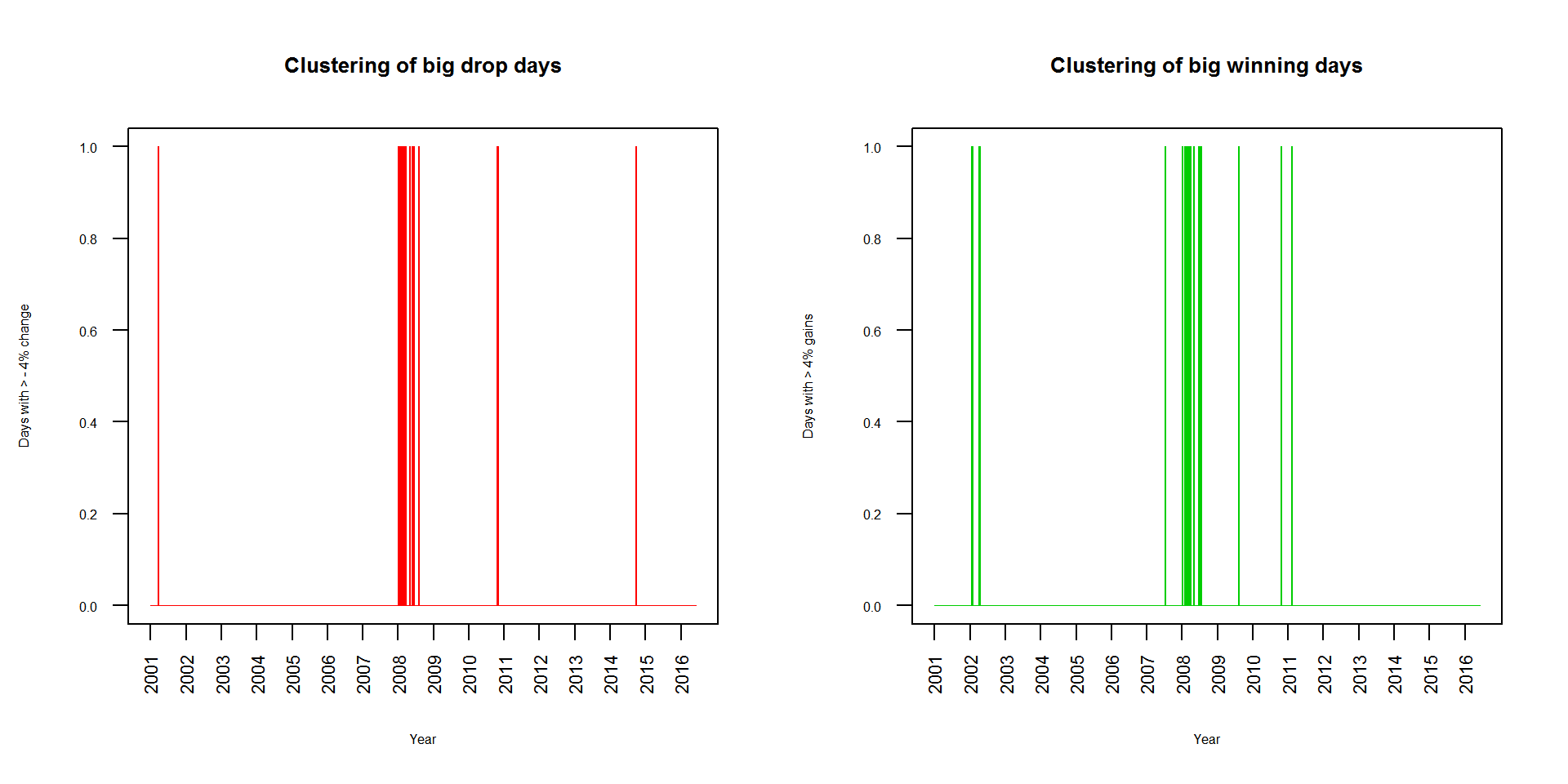Wow! Huge spike around 2008 - 2009. How much clustering is there? sum(VTI["2008/2009"]$InterDay < - 4) # Total number of drops > -4%
##  22
# As a percentage of all losing days...
sum(VTI["2008/2009"]$InterDay < - 4) / sum(VTI$InterDay < -4) 
##  0.7857143

What about wins during the same period?

sum(VTI["2008/2009"]$InterDay > 4) # Total number of winning days > 4% ##  15 # As a percentage of all winning days... sum(VTI["2008/2009"]$InterDay >  4) / sum(VTI$InterDay > 4) ##  0.6521739 So, during the crisis there were $$22$$ “big losing” days, and $$15$$ “big winning days”. plot(VTI$InterDay["2008-09-01/2009-03-01"], las=2, cex.axis=.6, main="Interday changes during crisis")
abline(h = 0)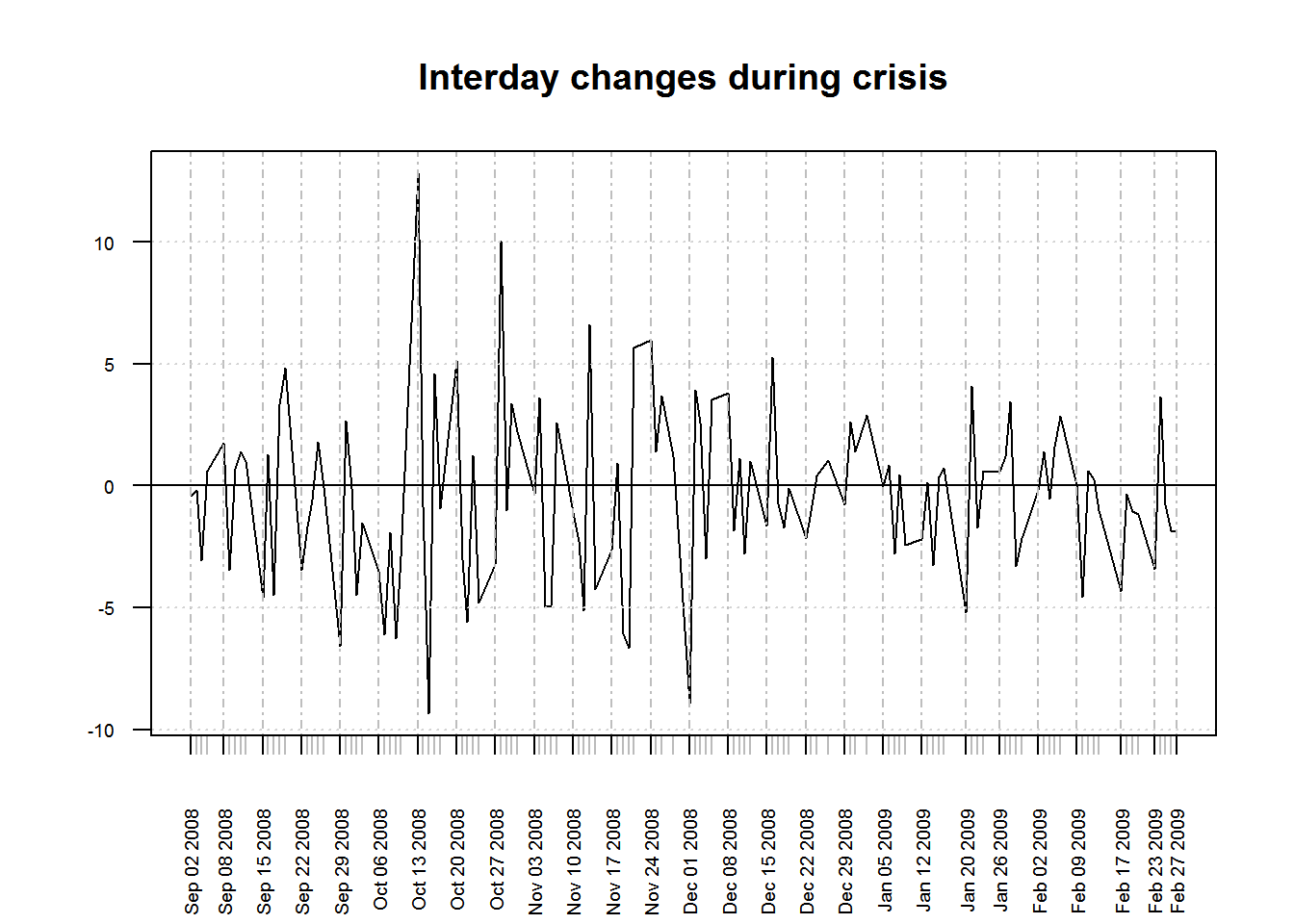Yet, the market tanked. Were the losing days worse, not just more numerous?

The maximum drop did occur during the crisis…

min(VTI["2008/2009"]$InterDay) ##  -9.352953 when? time(VTI["2008/2009"])[which.min(VTI["2008/2009"]$InterDay)]
##  "2008-10-15 EDT"

Here is the news flashback:

Another huge Dow loss Blue-chip indicator drubbed $$733$$ points - $$2$$nd biggest point loss ever - as recession fears resurface.

By Alexandra Twin, CNNMoney.com senior writer

Last Updated: October 15, 2008: 6:21 PM ET

NEW YORK (CNNMoney.com) – Recession talk scared Wall Street Wednesday, sending the Dow Jones industrial average to its second biggest one-day point loss ever.

A weak retail sales report and dour forecasts from the Federal Reserve, coupled with sober comments from Fed Chairman Ben Bernanke, sent stocks tumbling.

a whopping $$-9\%$$! Incredibly the maximum inter-day win also happen around this period…

max(VTI["2008/2009"]$InterDay) ##  12.82977 Wow! When did it happen? time(VTI["2008/2009"])[which.max(VTI["2008/2009"]$InterDay)]
##  "2008-10-13 EDT"
VTI["2008-10-13"]
##             Open  High   Low Close Adj.Close  Volume InterDay
## 2008-10-13 47.05 50.23 46.66 50.04     50.04 7777500 12.82977

and the news reflected it:

Dow jumps $$936$$ points and S&P up $$104$$, in the biggest point gains ever. The Dow, S&P and Nasdaq all gain over $$11\%$$.

By Alexandra Twin, CNNMoney.com senior writer

Last Updated: October 13, 2008: 6:15 PM ET

NEW YORK(CNNMoney.com)

Stocks rallied Monday afternoon, with the Dow rallying $$976$$ points during the session, as investors bet that the worst of the credit crisis is over, following a series of global initiatives announced over the last few days.

That bounce was predicated by the day’s news, with investors breathing a sigh of relief that some specifics were finally released regarding the $$\700$$ billion bank bailout.

Let’s hone down on the crisis period:

crisis = VTI["2008-09-01/2013-03-01"]
plot(time(crisis), crisis$InterDay < - 4, col=2, type='h', xlab="Year", ylab="", cex.axis=.5, cex.main=.8, cex.lab =.5,las=2, main = "Big losing and winning days during the crisis") points(time(crisis), crisis$InterDay > 4, col=3, type='h')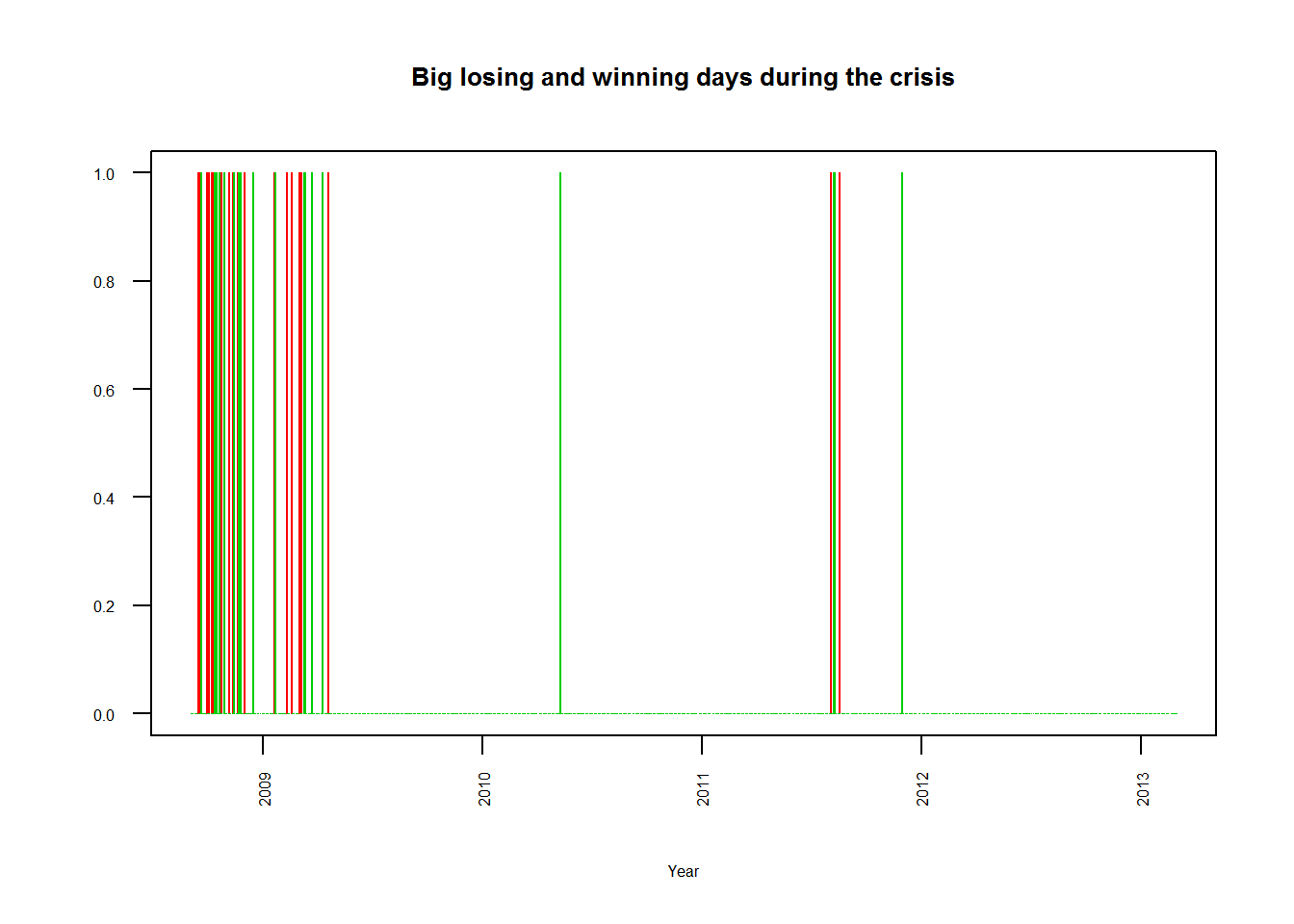Between the start of the crisis around September 2008, and the nadir, on March 09, 2009,

A year later, we know that March 9 was the bottom of a months-long financial panic that wiped away trillions of dollars in assets. But on what now appears to have been the best buying opportunity of a generation, many only wondered how much lower the markets would tumble. (Forbes)

For Dow, another $$12$$-year low.

S&P also finishes at lowest level in more than a decade as Wall Street resumes its retreat on economic worries.

By Alexandra Twin, CNNMoney.com senior writer Last Updated: March 9, 2009: 6:18 PM ET

there was a loss of

(drop = as.numeric(VTI$Adj.Close["2009-03-09"]) - as.numeric(VTI$Adj.Close["2008-09-10"]))
##  -28.22
drop/as.numeric(VTI$Adj.Close["2008-09-10"]) * 100 # In percentage... ##  -45.57493 which didn’t recover fully until March 2013, four years later: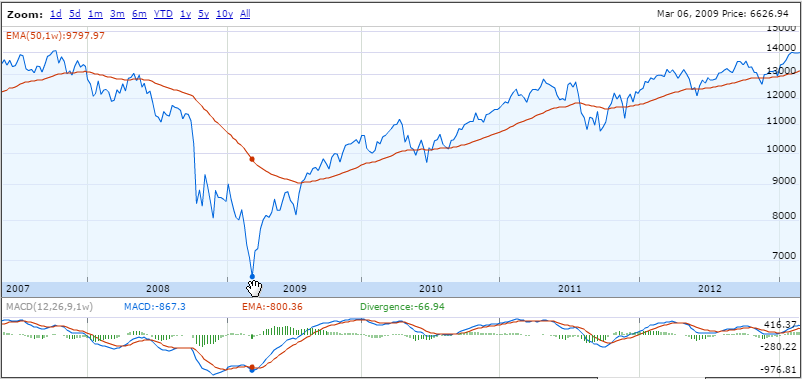What happened on October 10, 2009? Not much, it was Saturday. And on Monday VTI["2009-10-12"] ## Open High Low Close Adj.Close Volume InterDay ## 2009-10-12 54.63 54.84 54.38 54.6 54.6 868000 0.3676397 not much happened, either… October 14, 2009, perhaps? VTI["2009-10-14"] ## Open High Low Close Adj.Close Volume InterDay ## 2009-10-14 55.11 55.48 54.87 55.42 55.42 1322200 1.781445 Finally, let’s take a look at the fat tails of VTI as representative of the market, and as compared to the normal distribution: hist(VTI$InterDay,main="INTERDAY VTI % CHANGES: FAT TAILS",sub="fitted normal (in purple); pdf estimate (in blue)",xlab = "PERCENTAGE CHANGE FROM DAY TO DAY",
ylab = "FREQUENCY", prob = TRUE, col ="coral", ylim=c(0,0.5),
xlim=c(-4,4), cex.main=.9, cex.sub=.8, cex.lab=.7, border=F,
breaks = 33)

lines(density(VTI$InterDay,adjust=7),col="darkred",lwd=2) #Prettier, adjusted pdf estimate sd = sd(VTI$InterDay)
m = mean(VTI$InterDay) curve(dnorm(x,mean=m,sd=sd),col="grey60",lwd=2,add=T,yaxt="n") lines(density(VTI$InterDay,adjust=7),col="darkred",lwd=2) #Prettier, adjusted pdf estimate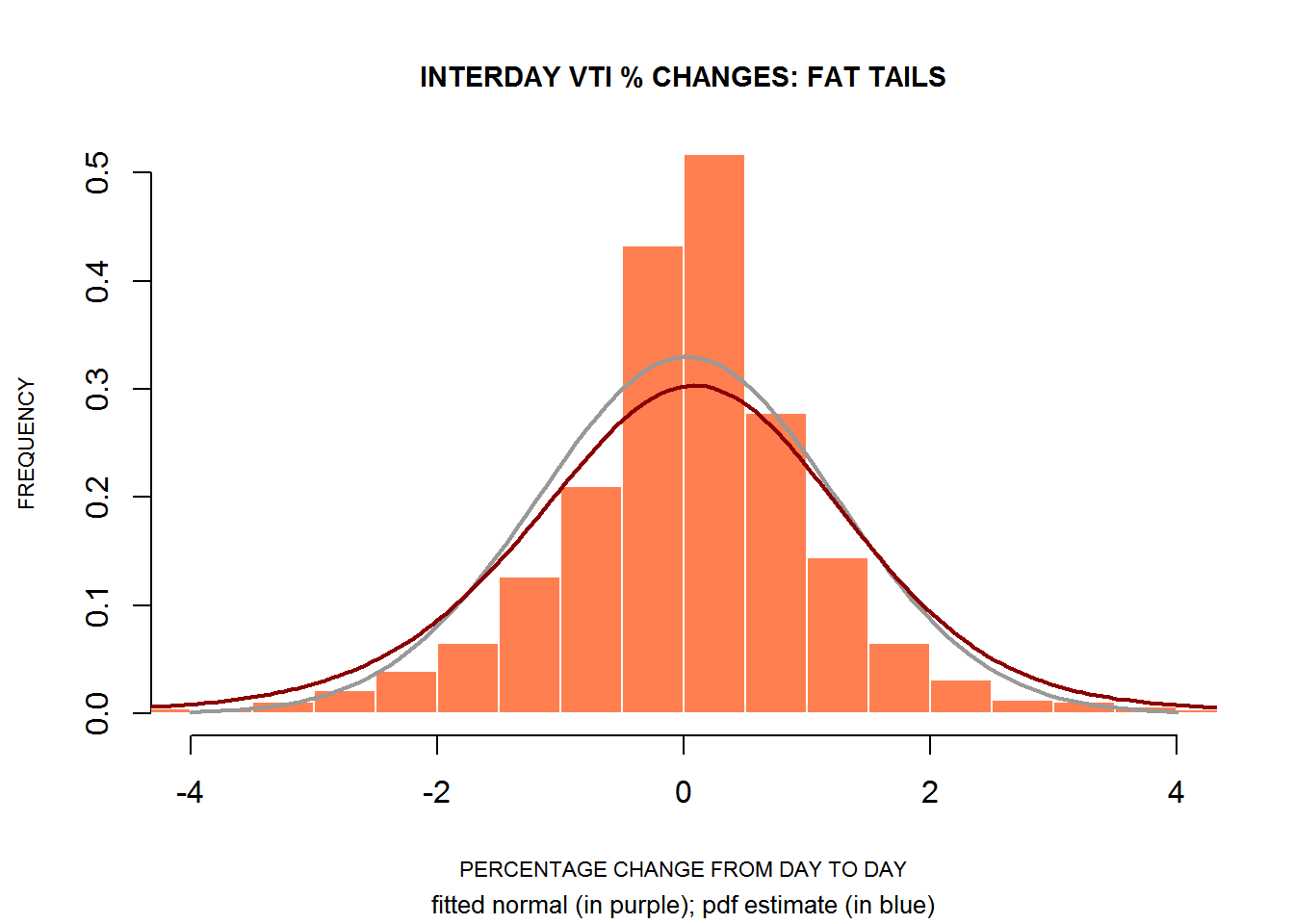But let’s objectivize the lack of normality:

qqnorm(VTI$InterDay, pch=19, cex=.1, col=rgb(0,0,1,0.5), cex.main=.8, cex.lab=.7, cex.axis=.7) qqline(VTI$InterDay, col=rgb(0,0,1,0.8))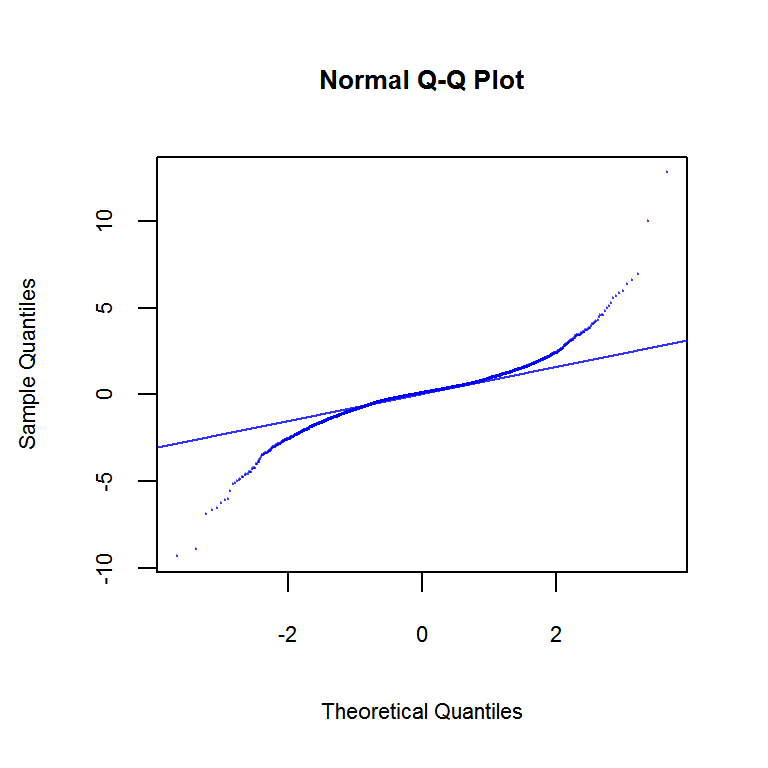shapiro.test(as.vector(VTI$InterDay)) ## ## Shapiro-Wilk normality test ## ## data: as.vector(VTI$InterDay)
## W = 0.90896, p-value < 2.2e-16